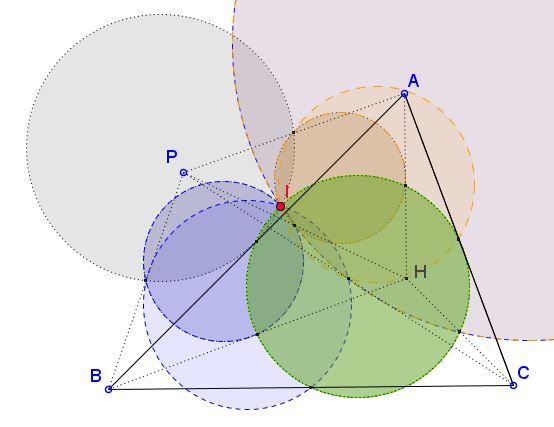# Concurrence of Ten Nine-Point Circles

### Problem

Given point $P$ in the plane of $\Delta ABC,$ let $H$ be the orthocenter of the triangles; consider three quadrilaterals $ABPH,$ $BCPH,$ and $ACPH.$ Each quadrilateral defines four triangles whose nine-point circles concur at a point, known as the Euler-Poncelet points of the quadrilateral.Prove that the three Euler-Poncelet points coincide.

### Solution

Solution is wanting; meanwhile you may wonder why the caption says "10 circles" instead of $4\times 3=12.$

Also, it has been observed that, similar to Griffiths' theorem, that point is the same for all points $P$ on a straight line through the circumcenter of $\Delta ABC.$

### Acknowledgment

The problem has been posted by Dao Thanh Oai at the CutTheKnotMath facebook page.

### Nine Point Circle